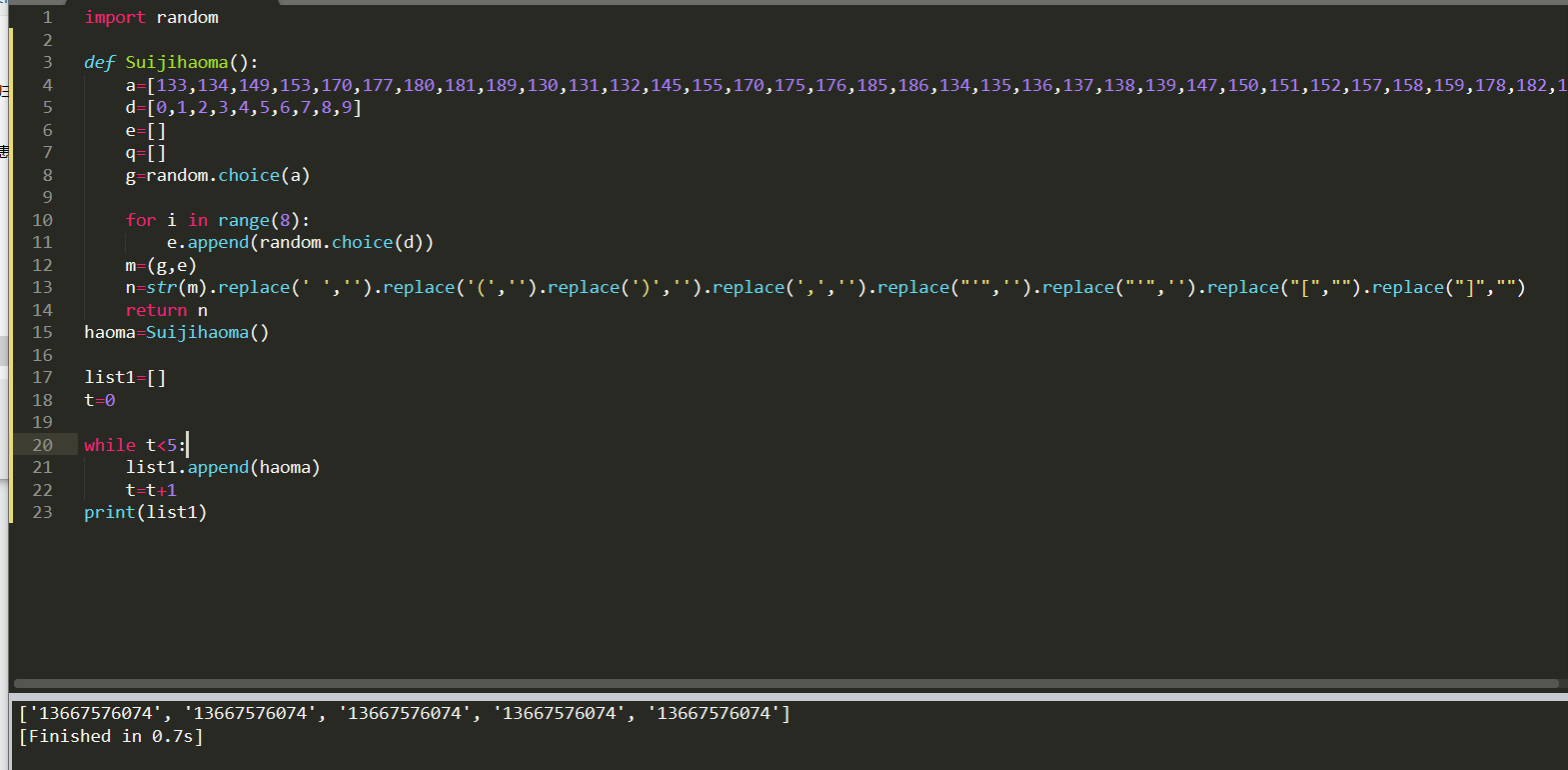2020-09-18 10:21

# python中怎样实现每循环一次就重新使用def方法一次

``````import random

def Suijihaoma():
a=[133,134,149,153,170,177,180,181,189,130,131,132,145,155,170,175,176,185,186,134,135,136,137,138,139,147,150,151,152,157,158,159,178,182,183,184,187,188]
d=[0,1,2,3,4,5,6,7,8,9]
e=[]
q=[]
g=random.choice(a)

for i in range(8):
e.append(random.choice(d))
m=(g,e)
n=str(m).replace(' ','').replace('(','').replace(')','').replace(',','').replace("'",'').replace("'",'').replace("[","").replace("]","")
return n
haoma=Suijihaoma()

list1=[]
t=0

while t<5:
list1.append(haoma)
t=t+1
print(list1)
``````• 点赞
• 写回答
• 关注问题
• 收藏
• 复制链接分享
• 邀请回答

#### 3条回答

• 把haoma=Suijihaoma()放在while内部，放在外部只调用一次，放在里面，每次都执行

点赞 1 评论 复制链接分享
•回答这么多问题就耍赖把我的积分一笔勾销了 7月前

电话号码是字符串，你非要用数字，你这么写从根本上就是错的。

点赞 2 评论 复制链接分享
• 生成号码的方法有很多，题主的方法也是可行的，只是效率有点低，代码有点凌乱。顺着题主的思路，我整理了一下，仅供参考。

``````>>> import random
>>> def mobile():
choices = (133,134,149,153,170) # 此处仅列出部分前缀
prefix = str(random.choice(choices))
return prefix + ''.join([str(random.randint(0,9)) for i in range(8)])

>>> mobiles = [mobile() for i in range(5)]
>>> mobiles
['15395255987', '15338347860', '13430999913', '17020377203', '15356104067']``````
点赞 评论 复制链接分享